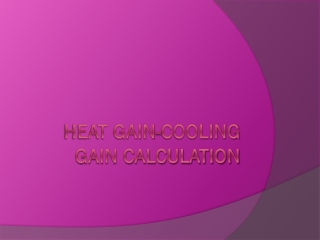DownloadDownload PresentationHEAT GAIN-COOLING GAIN CALCULATION

# HEAT GAIN-COOLING GAIN CALCULATION

Télécharger la présentation## HEAT GAIN-COOLING GAIN CALCULATION

- - - - - - - - - - - - - - - - - - - - - - - - - - - E N D - - - - - - - - - - - - - - - - - - - - - - - - - - -
##### Presentation Transcript

1. HEAT GAIN-COOLING GAIN CALCULATION

2. Each of the steps below is keyed to an example of a 30' long by 25' wide by 10' high elementary classroom space with 20 students (See diagram). Other pertinent information for this example are given as follows: a) Interior design temperature: 78ºF b) Exterior design temperature: 95ºF c) Exterior design humidity: 80%

3. Use the simplified design method utilized the space floor area to estimate the space cooling load in terms of supply air required. This accounts for the heat gains from people, equipment, lights, and building envelope.

4. a. Determine the floor area (ft2) for each space 30' x 25' = 750 SF b. Estimate the CFM air requirement Floor area x Cooling load factor = 750 SF x 1.5 CFM/SF = 1,125 CFM c. Calculate the total space cooling load - Assume the temperature at the cooling coil to be 55ºF. QS = 1.08 x CFM x ΔT (Interior Design Temp – Cooling Coil Temp) = 1.08 x 1,125 CFM x (78ºF – 55ºF) = 27,945 Btu/hr

5. Fresh air has both sensible and latent heat gain components. a. Estimate the occupancy – actual or by chart (see above Table 2) Estimate the number of people that will be in the room during normal use. For the classroom example, this would be 20 persons (actual occupancy). b. Estimate the CFM ventilation requirement The required rate of ventilation is 15 CFM per person. Therefore, for this classroom, 15 CFM/person x 20 person = 300 CFM of fresh air is required.

6. c. Calculate the sensible heat gains from ventilation Qsensible = 1.08 x CFM x ΔT (Outdoor Design Temp – Cooling Coil Temp) = 1.08 x 300 CFM x (95ºF – 55ºF) = 12,960 Btu/hr d. Calculate the latent heat gains from ventilation To determine latent heat gains from ventilation, the outside air temperature and relative humidity needs to be plotted on the psychrometric chart to determine the amount of moisture that must be removed from the air at the cooling coil. This is the change in moisture or the Δw.

7. Qlatent = 4840 x CFM x Δw = 4840 x 300 CFM x (0.029 – 0.075) = 31,218 Btu/hr • Total Ventilation Load QV = Qsensible + Qlatent (Btu/hr) = 12,960 +31,218 = 44,178 Btu/hr

8. Total CFM Air Requirement Total Space Cooling Load CFM + Total Ventilation Load CFM = Total Cooling Load CFM = 1,125 + 300 = 1,425 • Total Cooling Required = Total Space Cooling Load + Total Ventilation Load = QS (Btu/hr) + QV (Btu/hr) = QTotal (Btu/hr) = 27,945 + 44,178 = 72,123 Btu/hr